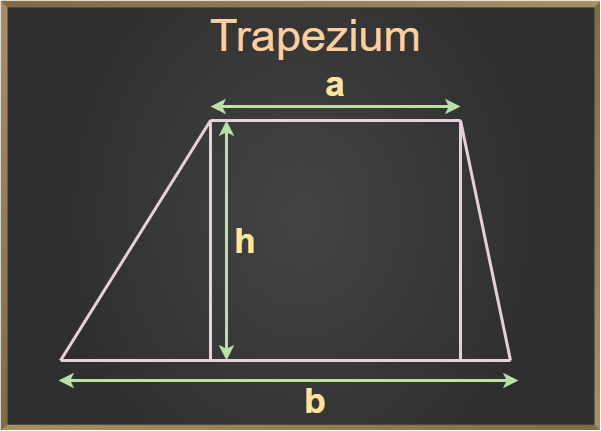GeeksforGeeks App
Open AppBrowser
Continue

# How to find the distance between parallel sides of a trapezium?

A trapezium is a quadrilateral with one pair of parallel opposite sides, according to Euclidean geometry. A trapezium is derived from the Greek word “trapeza,” which means “table.” It is a two-dimensional quadrilateral (made up of four straight lines) with two parallel opposite sides. The opposite parallel sides are referred to as the trapezium’s base, while the non-parallel sides are referred to as its legs. It has four sides and four corners and is a closed plane shape. It is also called a trapezoid.

Since a trapezium is a quadrilateral, it has 4 different sides and 4 different edges. The measures of all 4 internal angles of a trapezium always sum up to 360°.

## Area of a Trapezium

The area of any closed figure is the space that this object encloses. It is the region covered by a trapezium in a two-dimensional plane. It is the space bounded by two dimensions and measured in square units. Thus, the area of trapezium is given by,

Area = 1/2 × Sum of parallel sides × Distance between them

Let a and b be the parallel sides of a given trapezium ABCD and h be the distance between a and b. Then, the above formula can be simplified as:Area of trapezium = 1/2 × (a+b) × h

The area of a trapezium is denoted in square units.

## How to find the Distance Between Parallel Sides of a Trapezium?

Solution:

Since the formula for the area of a trapezium uses the sum of parallel sides and the distance between them, it implies that if any of the two quantities is given, the third can be computed conveniently. Thus, if area and the sum of parallel sides of a trapezium is given, the distance between them can be calculated by substituting the given values in the formula for the area of a trapezium.

Example: Suppose a trapezium ABCD with an area of 350 cm2 has parallel sides of the measures 25 cm and 10 cm respectively. Then, its height, i.e. the distance between its parallel sides can be calculated by substituting the given values in the formula for the area of a trapezium.

Solution:

Area = 1/2×[(sum of parallel sides)×(height of trapezium)]

Area = 1/2×(25 + 10)×H

350  = 1/2×35×H

H = 20 cm

## Solved Examples

Example 1: Find the height of a trapezium whose parallel sides sum up to 100 cm and area is 2500 cm2.

Solution:

Area of the trapezium = 2500 cm2

Sum of the parallel sides of the trapezium = 100 cm

As we know that

Area of trapezium = 1/2 × (Sum of the parallel sides) × Height

2500 = 1/2 × 100 × Height

Height = (2500 × 2)/100

Height = 50 cm

Therefore,

Height of the trapezium is 50 cm.

Example 2: Find the sum of parallel sides of a trapezium whose height is 60 cm and the area is 1200 cm2.

Solution:

Area of the trapezium = 1200 cm2

Height of the trapezium = 60 cm

As we know that,

Area of trapezium = 1/2 × (Sum of the parallel sides) × Height

1200 = 1/2 × (Sum of the parallel sides) × 60

Sum of the parallel sides = (1200 × 2)/60

Sum of the parallel sides = 40 cm

Example 3: Find the area of the trapezium with the sum of the parallel sides of 70 m and the height of 10 m.

Solution:

Sum of the parallel sides = 70 m

Height of the trapezium = 10 m

As we know that,

Area of trapezium = 1/2 × (Sum of the parallel sides) × Height

Area of trapezium = 1/2 × (70) × 10

Area of trapezium = 350 m2

Therefore,

Area of trapezium is 350 m2.

Example 4: If the sum of the parallel sides of the trapezium is twice the height and the area of the trapezium is 900 m2. Then find the sum of the parallel sides of the trapezium and its height.

Solution:

Area = 900 m2

Sum of the parallel sides of the trapezium is double its height

Assume,

Height of the trapezium = x, than

Sum of the parallel sides of the trapezium = 2x

As we know that,

Area of trapezium = 1/2 × (Sum of the parallel sides) × Height

900 = 1/2 × 2x × x

900 = 1/2 × 2x2

900 = x2

x = √900

x = 30 m

Thus,

Height of the trapezium = x = 30 m

Sum of the parallel sides of the trapezium = 2x = 2 × 30 = 60 m

## FAQs on Trapezium

Question 1: What is a trapezium?

Solution:

A trapezium shape is a two-dimensional quadrilateral with a pair of non-adjacent parallel sides and a pair of non-parallel sides. It appears to be a triangle cut from the top.

Question 2. Is Trapezium a Quadrilateral?

Solution:

Trapezium is, indeed, a quadrilateral. It is composed of four sides, four vertices, and four angles. The sum of a trapezium’s four interior angles is 360 degrees.

Question 3. Is Trapezium a Parallelogram?

Solution:

Since it only has one pair of parallel sides, a trapezium is not a parallelogram. A parallelogram, on the other hand, has two pairs of parallel sides.

Question 4. What is Isosceles Trapezium?

Solution:

An isosceles trapezium has two legs or non-parallel sides that are equal to each other. The angles opposite those two equal sides are equal as well.

### Related Resources

My Personal Notes arrow_drop_up
Related Tutorials